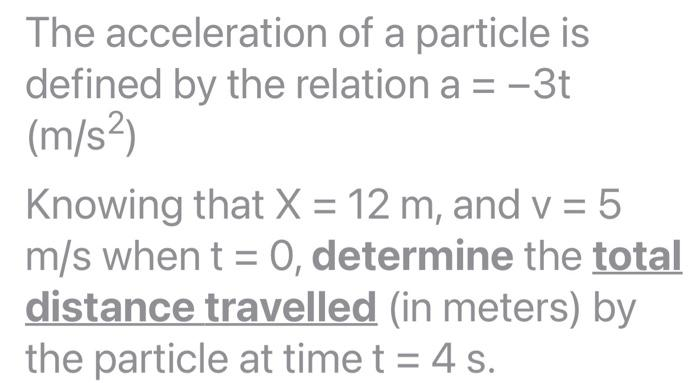# Question Solved1 AnswerThe acceleration of a particle is defined by the relation a = -3t (m/s) Knowing that X = 12 m, and v = 5 m/s when t = 0, determine the total distance travelled (in meters) by the particle at time t = 4 s.RGFJ5L The Asker · Mechanical EngineeringTranscribed Image Text: The acceleration of a particle is defined by the relation a = -3t (m/s) Knowing that X = 12 m, and v = 5 m/s when t = 0, determine the total distance travelled (in meters) by the particle at time t = 4 s.
More
Transcribed Image Text: The acceleration of a particle is defined by the relation a = -3t (m/s) Knowing that X = 12 m, and v = 5 m/s when t = 0, determine the total distance travelled (in meters) by the particle at time t = 4 s.